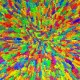# Adventures in R: Creating a Pseudo-CDF Plot for Binary Data

(Alternate title: “Ha, I’m Dumb”)
(Alternate alternate title: “Skip This if Statistics Bore You”)

You may recall a few days ago during one of my Blog Stats blogs I mentioned the problem of creating a cumulative distribution function-type plot for binary data, which would show the cumulative number of times one of the two binary variables occurred over some duration of another variable.

Um, let’s go to the actual example, ‘cause that description sucked.

Let’s say I have two variables called Blogs and Images for a set of data for which N = 2193. The variable Blogs gives the blog number for each post, so it runs from 1 to 2193. The variable Images is a binary variable and is coded 0 if the blog in question contains no image(s) and 1 if the blog contains 1 or more images.

Simple enough, right?

So what I was trying to do was create an easy-to-interpret visual that would show the increase in the cumulative number of blogs containing images over time, where time was measured by the Blogs variable.

Not being ultra well-versed in the world of visually representing binary data, this was the best I could come up with in the heat of the analysis:If you take a look at the y-axis, it becomes clear that due to the coding, the Images variable could only either equal 0 or 1. When it equaled 1, this plot drew a vertical black line at the spot on the x-axis that matched the corresponding Blogs variable. It’s not the worst graph (and if you scan it at the grocery store, you’ll probably end up with a bag of Fritos or something), but it’s not the easiest-to-interpret graph on the planet either, now is it?

What I was really looking for was some sort of cumulative distribution function (CDF) plot, but for binary data. I like how Wiki puts it: “Intuitively, [the CDF] is the “area so far” function of the probability distribution.” As you move right on the x-axis, the CDF curve lines up with the probability (given on the y-axis) that the variable, at that point on the x-axis, is less than or equal to the value indicated by the curve. Assuming your y-axis is set for probability (mine isn’t, but it’s still easy to interpret). This is all well and good for well-behaving ratio data, but what happens if I want to do such a plot for a dichotomously-coded variable?

1) Be a spazz and write some R code to get it done, or
2) Be an anti-spazz and look up if anybody’s written some R code to get it done.

I originally wanted to do A, which I did, but B was actually a lot harder than it should have been.

Let’s look at A first. I wanted to plot the number of surveys containing images against time, measured by the Blogs variable. Since I coded blogs containing images as 1 and blogs not containing images as 0, all I needed to get R to do was spit out a list of the cumulative sum of the Images variable at each instance of the Blogs variable (so a total of 2193 sums). Then plot it.

R and I have a…history when it comes to me attempting to write “for” loops. But it finally worked this time. I’ll just give you that little segment, ‘cause the rest of the code’s for the plotting parameters and too long/bothersome to throw on here.

```for (m in (1:length(ximage))){
newimage=ximage[1:m]
xnew=sum(newimage)
t=cbind(m,xnew)
points(t,type="h",pch="1")
}```

ximage is the name of the vector containing the coded Images variable. So what this little “for” loop does is create a new variable (newimage) for every vector length between 1 and 2193 instances of the Images variable. Another new variable (xnew) calculated the sum of 1s in each newimage. t combines the Blogs number (1 through 2193) with the matching xnew. Finally, the points of t are plotted (on a pre-created blank plot).

So. Wanna see?Woo!

So I actually figured this out on Wednesday, but I didn’t blog about it because I wanted to see if I could find a function that already does what I wanted. Why did it take an extra three days to find it? Because I couldn’t for the life of me figure out what that type of plot was called. It’s not a true CDF because it’s not a continuous variable we’re dealing with. But after obsessively searching (this is the reason for the alternate title—I should have known what this type of plot was called), I finally found a (very, very simple) function that makes what this is: a cumulative frequency graph (I know, I know, duh, right?).

So here’s the miniscule little bit of code needed to do what I did:

```cumfreq=cumsum(ximage)
plot(cumfreq, type="h")```

The built-in function (it was even in the damn base package. SHAME, Claudia, SHAME!!) cumsum gives a vector of the sum at each instance of ximage; plotting that makes the exact same graph as my code (except I manually fancied up my axes in my code).Cool, eh?

Maybe I’ll post my full code once I make it uncustomized to this particular problem.

### One response

1.[…] Adventures in R: Creating A Pseudo-CDF Plot for Binary Data […]

Like